Helpers for working with DICOM files

Patching

get_dicom_files[source]

get_dicom_files(path, recurse=True, folders=None)

Get dicom files in path recursively, only in folders, if specified.

Open a DICOM file

fastai.medical.imaging uses pydicom.dcmread to read a DICOM file. To view the header of a DICOM, specify the path of a test file and call dcmread.

TEST_DCM = Path('images/sample.dcm')
dcm
Dataset.file_meta -------------------------------
(0002, 0000) File Meta Information Group Length  UL: 176
(0002, 0001) File Meta Information Version       OB: b'\x00\x01'
(0002, 0002) Media Storage SOP Class UID         UI: CT Image Storage
(0002, 0003) Media Storage SOP Instance UID      UI: 9999.180975792154576730321054399332994563536
(0002, 0010) Transfer Syntax UID                 UI: Explicit VR Little Endian
(0002, 0012) Implementation Class UID            UI: 1.2.40.0.13.1.1.1
(0002, 0013) Implementation Version Name         SH: 'dcm4che-1.4.38'
-------------------------------------------------
(0008, 0018) SOP Instance UID                    UI: ID_e0cc6a4b5
(0008, 0060) Modality                            CS: 'CT'
(0010, 0020) Patient ID                          LO: 'ID_a107dd7f'
(0020, 000d) Study Instance UID                  UI: ID_6468bdd34a
(0020, 000e) Series Instance UID                 UI: ID_4be303ae64
(0020, 0010) Study ID                            SH: ''
(0020, 0032) Image Position (Patient)            DS: [-125.000, -122.268, 115.936]
(0020, 0037) Image Orientation (Patient)         DS: [1.000000, 0.000000, 0.000000, 0.000000, 0.978148, -0.207912]
(0028, 0002) Samples per Pixel                   US: 1
(0028, 0004) Photometric Interpretation          CS: 'MONOCHROME2'
(0028, 0010) Rows                                US: 256
(0028, 0011) Columns                             US: 256
(0028, 0030) Pixel Spacing                       DS: [0.488281, 0.488281]
(0028, 0100) Bits Allocated                      US: 16
(0028, 0101) Bits Stored                         US: 16
(0028, 0102) High Bit                            US: 15
(0028, 0103) Pixel Representation                US: 1
(0028, 1050) Window Center                       DS: "40.0"
(0028, 1051) Window Width                        DS: "100.0"
(0028, 1052) Rescale Intercept                   DS: "-1024.0"
(0028, 1053) Rescale Slope                       DS: "1.0"
(7fe0, 0010) Pixel Data                          OW: Array of 131072 elements
type(dcm)
pydicom.dataset.FileDataset

classTensorDicom[source]

TensorDicom(x, **kwargs) :: TensorImage

Inherits from TensorImage and converts the pixel_array into a TensorDicom

classPILDicom[source]

PILDicom() :: PILBase

This class represents an image object. To create :py:class:~PIL.Image.Image objects, use the appropriate factory functions. There's hardly ever any reason to call the Image constructor directly.

• :py:func:~PIL.Image.open
• :py:func:~PIL.Image.new
• :py:func:~PIL.Image.frombytes

None[source]

dcm.pixels
tensor([[-1024., -1024., -1024.,  ..., -1024., -1024., -1024.],
[-1024., -1024., -1024.,  ..., -1024., -1024., -1024.],
[-1024., -1024., -1024.,  ..., -1024., -1024., -1024.],
...,
[-1024., -1024., -1024.,  ..., -1024., -1024., -1024.],
[-1024., -1024., -1024.,  ..., -1024., -1024., -1024.],
[-1024., -1024., -1024.,  ..., -1024., -1024., -1024.]])

None[source]

scaled_px uses RescaleSlope and RescaleIntercept values to correctly scale the image so that they represent the correct tissue densities. You can observe what scaled_px does by viewing the the pixel distribution of a dicom image. The histogram below displays the current pixel distribution which shows a pixel range between -1133 and 2545.

plt.hist(dcm.pixels.flatten().numpy());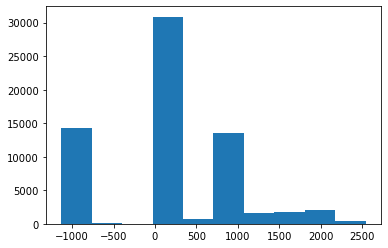As shown in the header of the test image the RescaleIntercept has a value of -1024.0 and a RescaleSlope value of 1.0. scaled_px will scale the pixels by these values.

plt.hist(dcm.scaled_px.flatten().numpy());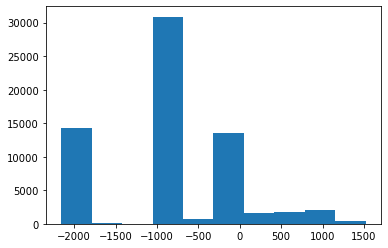The pixel distibution is now between -2157 and 1521

array_freqhist_bins[source]

array_freqhist_bins(n_bins=100)

A numpy based function to split the range of pixel values into groups, such that each group has around the same number of pixels

Tensor.freqhist_bins[source]

Tensor.freqhist_bins(n_bins=100)

A function to split the range of pixel values into groups, such that each group has around the same number of pixels

For example with n_bins set to 1 this means the bins will be split into 3 distinct bins (the beginning, the end and the number of bins specified by n_bins.

t_bin = dcm.pixels.freqhist_bins(n_bins=1)
t_bin
tensor([-1076.,    40.,  2375.])
plt.hist(t_bin.numpy(), bins=t_bin, color='c')
plt.plot(t_bin, torch.linspace(0,1,len(t_bin)));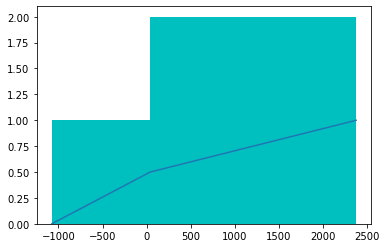with n_bins at 100

t_bin = dcm.pixels.freqhist_bins(n_bins=100)
t_bin
tensor([-1076., -1026., -1024., -1021.,    28.,    30.,    31.,    32.,    33.,
34.,    35.,    36.,    37.,    38.,    39.,    40.,    41.,    42.,
44.,    48.,    52.,    58.,    66.,    72.,    76.,    80.,    85.,
91.,    94.,    98.,   103.,   111.,   123.,   161.,   219.,   478.,
829.,   999.,  1027.,  1038.,  1044.,  1047.,  1049.,  1050.,  1051.,
1052.,  1053.,  1054.,  1055.,  1056.,  1057.,  1058.,  1059.,  1060.,
1062.,  1066.,  1108.,  1265.,  1453.,  1616.,  1741.,  1838.,  1943.,
2051.,  2220.,  2375.])
plt.hist(t_bin.numpy(), bins=t_bin, color='c'); plt.plot(t_bin, torch.linspace(0,1,len(t_bin)));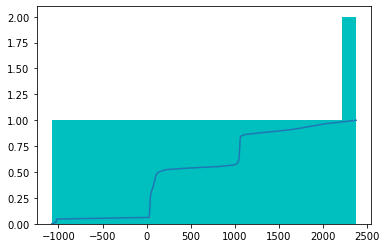Tensor.hist_scaled_pt[source]

Tensor.hist_scaled_pt(brks=None)

Tensor.hist_scaled[source]

Tensor.hist_scaled(brks=None)

Scales a tensor using freqhist_bins to values between 0 and 1

The test image has pixel values that range between -1000 and 2500

plt.hist(dcm.pixels.flatten().numpy(), bins=100);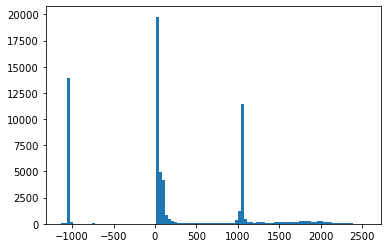hist_scaled provides a way of scaling the input pixel values to between 0 and 1

tensor_hists = dcm.pixels.hist_scaled()
plt.hist(tensor_hists.flatten().numpy(), bins=100);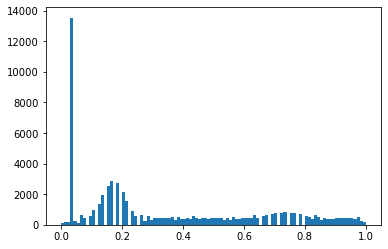Dataset.hist_scaled[source]

Dataset.hist_scaled(brks=None, min_px=None, max_px=None)

Pixels scaled to a min_px and max_px value

data_scaled = dcm.hist_scaled()
plt.imshow(data_scaled, cmap=plt.cm.bone);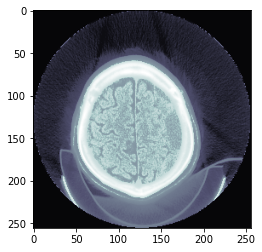data_scaled = dcm.hist_scaled(min_px=100, max_px=1000)
plt.imshow(data_scaled, cmap=plt.cm.bone);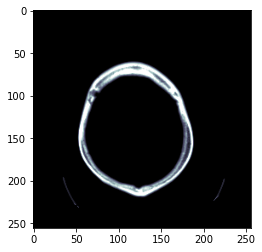Dicom images can contain a high amount of voxel values and windowing can be thought of as a means of manipulating these values in order to change the apperance of the image so particular structures are highlighted. A window has 2 values:

• l = window level or center aka brightness
• w = window width or range aka contrast

Tensor.windowed[source]

Tensor.windowed(w, l)

Scale pixel intensity by window width and window level

Dataset.windowed[source]

Dataset.windowed(w, l)

plt.imshow(dcm.windowed(*dicom_windows.brain), cmap=plt.cm.bone);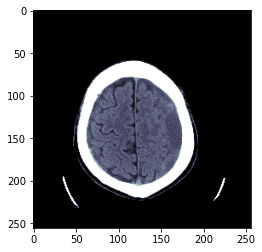classTensorCTScan[source]

TensorCTScan(x, **kwargs) :: TensorImageBW

Inherits from TensorImageBW and converts the pixel_array into a TensorCTScan

tensor_ct = TensorCTScan(dcm.pixel_array)
tensor_ct.show();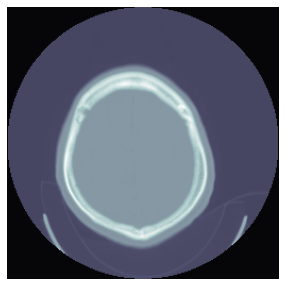classPILCTScan[source]

PILCTScan() :: PILBase

This class represents an image object. To create :py:class:~PIL.Image.Image objects, use the appropriate factory functions. There's hardly ever any reason to call the Image constructor directly.

• :py:func:~PIL.Image.open
• :py:func:~PIL.Image.new
• :py:func:~PIL.Image.frombytes

Dataset.show[source]

Dataset.show(frames=1, scale=True, cmap=<matplotlib.colors.LinearSegmentedColormap object at 0x7fc5dc98c370>, min_px=-1100, max_px=None, **kwargs)

Adds functionality to view dicom images where each file may have more than 1 frame

scales = False, True, dicom_windows.brain, dicom_windows.subdural
titles = 'raw','normalized','brain windowed','subdural windowed'
for s,a,t in zip(scales, subplots(2,2,imsize=4).flat, titles):
dcm.show(scale=s, ax=a, title=t)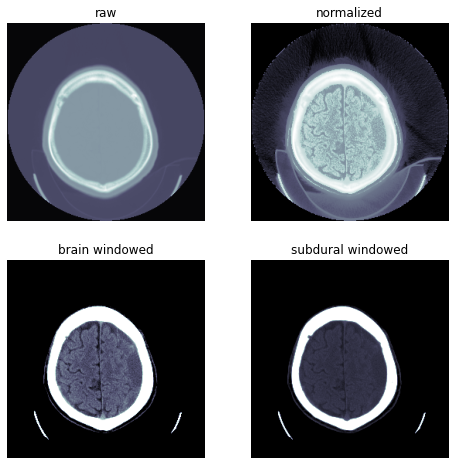dcm.show(cmap=plt.cm.gist_ncar, figsize=(6,6))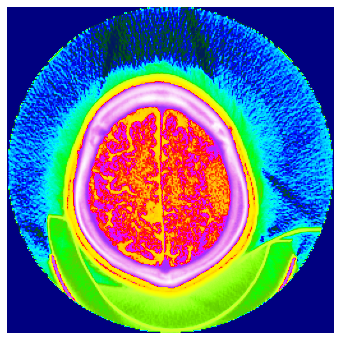Some dicom datasets such as the The Thyroid Segmentation in Ultrasonography Dataset is a dataset where each image has multiple frames per file (hundreds in this case). By default the show function will display 1 frame but if the dataset has multiple frames you can specify the number of frames to view.

Dataset.show[source]

Dataset.show(frames=1, scale=True, cmap=<matplotlib.colors.LinearSegmentedColormap object at 0x7fc5dc98c370>, min_px=-1100, max_px=None, **kwargs)

Adds functionality to view dicom images where each file may have more than 1 frame

dcm.show()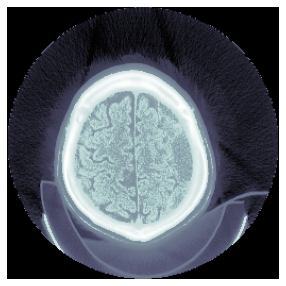Dataset.pct_in_window[source]

Dataset.pct_in_window(dcm:Dataset, w, l)

% of pixels in the window (w,l)

dcm.pct_in_window(*dicom_windows.brain)
0.19049072265625

pct_in_window can be used to check what percentage of the image is composed of meaningful pixels (pixels within the specified window)

uniform_blur2d[source]

uniform_blur2d(x, s)

Uniformly apply blurring

ims = dcm.hist_scaled(), uniform_blur2d(dcm.hist_scaled(), 20), uniform_blur2d(dcm.hist_scaled(), 50)
show_images(ims, titles=('original', 'blurred 20', 'blurred 50'))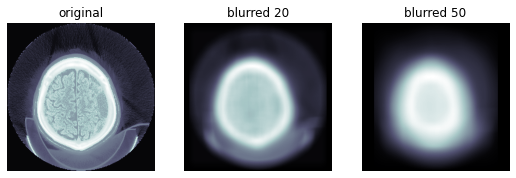gauss_blur2d[source]

gauss_blur2d(x, s)

Apply gaussian_blur2d kornia filter

ims = dcm.hist_scaled(), gauss_blur2d(dcm.hist_scaled(), 20), gauss_blur2d(dcm.hist_scaled(), 50)
show_images(ims, titles=('original', 'gauss_blur 20', 'gauss_blur 50'))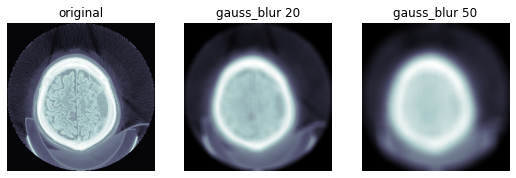Images are often affected by random variations in intensity values, called noise. Gaussian noise contains variatons in intensity that are drawn from a Gaussian or normal distribution. A Guassian filter is usually used to blur edges and remove smaller or thinner areas in order to preserve the most important information

Create a mask from the blurred image